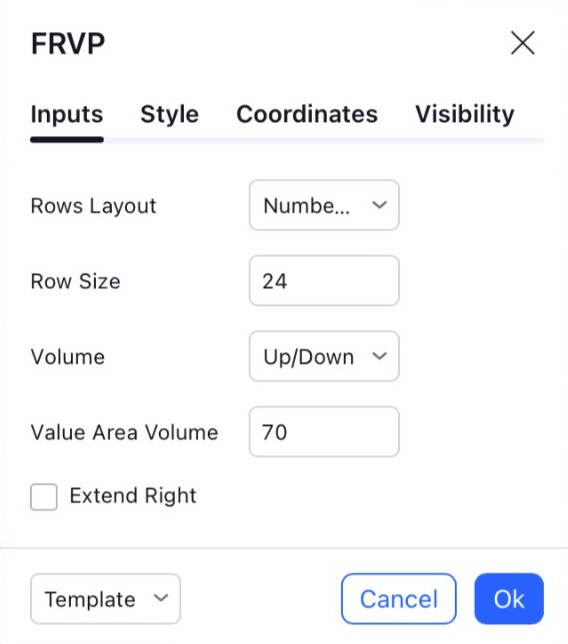# Fixed Range Volume Profile

#### Definition

Fixed Range Volume Profile (FRVP) indicator calculates volume data within a specified time period, allowing traders to analyse the volume activity of a particular range instead of the entire price movement of an asset.

Note: you can read more about traits shared by all Volume Profile indicators in the Volume Profile Indicators: basic concepts Help Center article. The text below describes features unique to this particular Volume Profile indicator.

#### Calculation

When calculating Volume Profile Fixed Range, we check a list of timeframes in a sequence until the number of bars in the time interval for which VP is calculated will be fewer than 5000. The sequence of timeframes is: 1, 3, 5, 15, 30, 60, 240, 1D. If the bars that the indicator covers amount in total to 5 minutes or fewer, or if it is used on any second-based timeframe, the indicator will take data from the 1S timeframe for its calculations, regardless of the number of bars covered.

Consider the following example. The VPFR indicator is set to calculate the profile for the period that starts on the first Monday bar and ends on the last Sunday bar of the same week. The symbol is traded 24x7 (for the sake of the experiment, let's assume there are no gaps where no trades were happening and each minute of every week has a bar associated with it).

1. First, the indicator checks the "1" timeframe, sees that the 1m chart for this symbol has 7D * 1440m = 10080 bars of 1m data, which is above the 5000 bar limit. It selects the next timeframe in the sequence.
2. Next, the indicator does the same with the "3" timeframe. The 3m chart for the symbol has 7D * (1440 / 3) = 3360 bars of data. This is below the 5000 bar limit, so the 3m bars will be used in the VPFR calculation.

Note: There is a limited number of lower timeframe bars available for the indicator's calculations. If the FRVP is set to be calculated very deep in the symbol's history, there might be no lower timeframe information for that period of time. In such cases, the Chart timeframe will be used for the calculation instead.

To demonstrate this, try adding FRVP to NASDAQ:AAPL, 1D, and setting it up to span a one-day period that starts on Dec 12, 1980. There won't be lower timeframe data for this time interval, so FRVP calculation will be carried out at the Chart resolution (1D).

#### Building on a single bar

Note that while building Fixed Range Volume Profile on a single bar, the close > open condition is used to calculate the Up/Down Volume. i.e., if close > open, the price movement is considered Up Volume, if close <= open, it's Down Volume.

#### Inputs##### Rows Layout / Row Size

These two inputs specify the way the indicator calculates how many rows each histogram will have.

If Rows Layout is set to “Number of Rows”, Row Size sets the total number of rows in the histogram: for example, with Row Size of 25 the histogram, top to bottom, will have 25 rows. The height of each row will be calculated automatically; in the example above, a Volume Profile histogram spanning the price from 200 to 300 will have (300 - 200) / 25 = 4 points per each row.

If this input is set to “Ticks Per Row”, Row Size indicates the number of ticks in each single row (1 tick being the minimal move the price for this symbol can make: for example, for NASDAQ:AAPL, 1 tick is 0.01 because the price needs to move at least 0.01 USD, i.e. 1 cent). E.g., setting it to 25 will mean that each row has the height of 25 ticks. In that case, the number of rows will be calculated automatically, and the Volume Profile histogram spanning the price from 200 to 300 for a symbol with a mintick of 0.01 will have (300 - 200) / 0.01 / 25 = 400 rows per each histogram.

##### Volume

Toggles between showing total volume for each row (Total), splitting each row into up/down (Up/Down), or showing their difference (Delta).

##### Value Area Volume

Specifies what percentage of all volume for the trading session should be highlighted by Value Area.

##### Extend Right

The Extend Right parameter allows one to continue building the profile, including all historical candles and all new emerging candles to the right of the second coordinate.

#### Coordinates

#1 (bar) - sets the position of the left border of the Volume Profile. The VP will start before this position.

#2 (bar) - sets the position of the right border of the Volume Profile. The VP will end after this position.